Function Repository Resource:

# FourPointParabolas

Get the parabolas that go through four points

Contributed by: Ed Pegg Jr
 ResourceFunction["FourPointParabolas"][pts,{x,y}] returns equations in the variables x and y for the parabolas that go through four given 2D points pts. ResourceFunction["FourPointParabolas"][pts] uses the formal variables x and y.

## Details

Isaac Newton explicitly constructed 4-point parabolas in Arithmetica Universalis (1707).
The general form of a parabola is A+B x+C y+(D x+y)2 = 0. This shows that four data points are required to specify a parabola. Since the equation is quadratic it is satisfied by two parabolas (though possibly with complex coefficients).

## Examples

### Basic Examples (4)

Find parabolas through four points:

 In:=Out=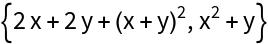Plot the parabolas:

 In:=Out=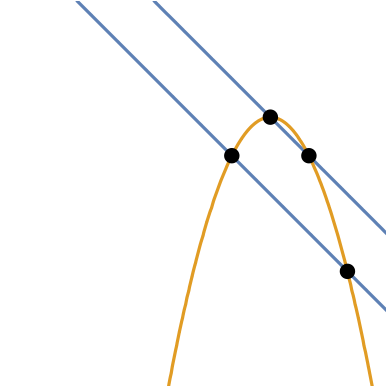Find parabolas through four points:

 In:=Out=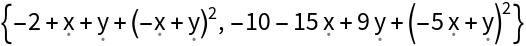Plot the parabolas:

 In:=Out=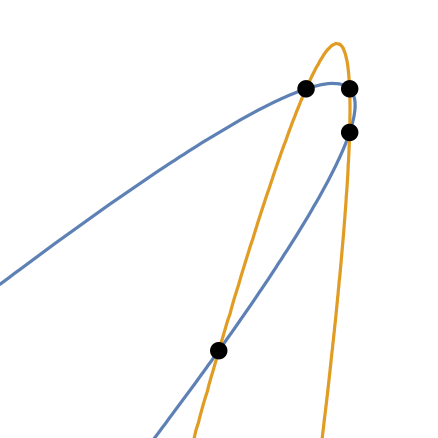Recover the points by setting the equations equal to each other and zero:

 In:=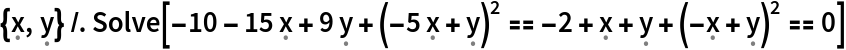Out=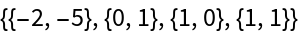Find parabolas through four points:

 In:=Out=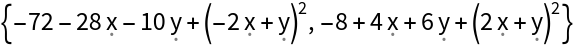Plot the parabolas:

 In:=Out=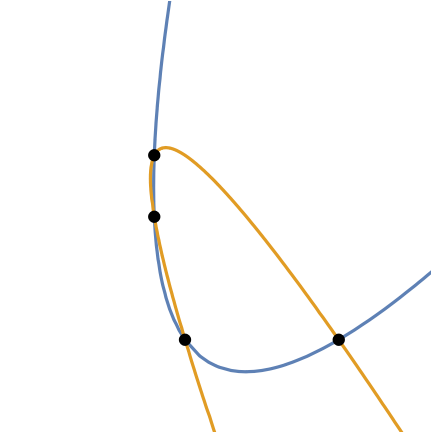Find parabolas through four points:

 In:=Out=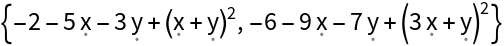Plot the parabolas:

 In:=Out=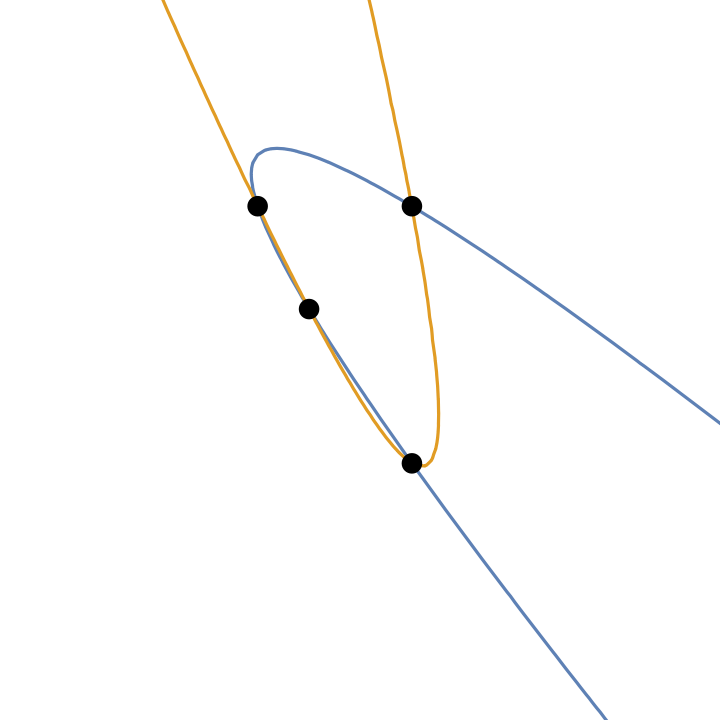### Possible Issues (2)

Some point sets do not return real-valued parabolas:

 In:=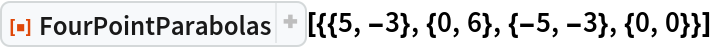Out=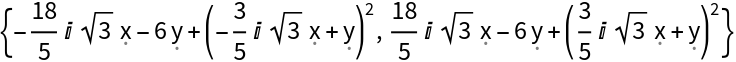When sets of points define parallel lines, the parabolas are degenerate:

 In:=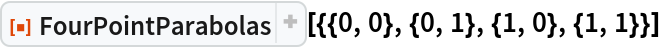Out=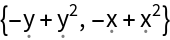### Neat Examples (2)

For random, uniformly-distributed points in a rectangle, the probability of a hyperbola is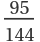and the probability of an ellipse is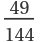. This distribution can be seen by drawing the parabolas:

 In:=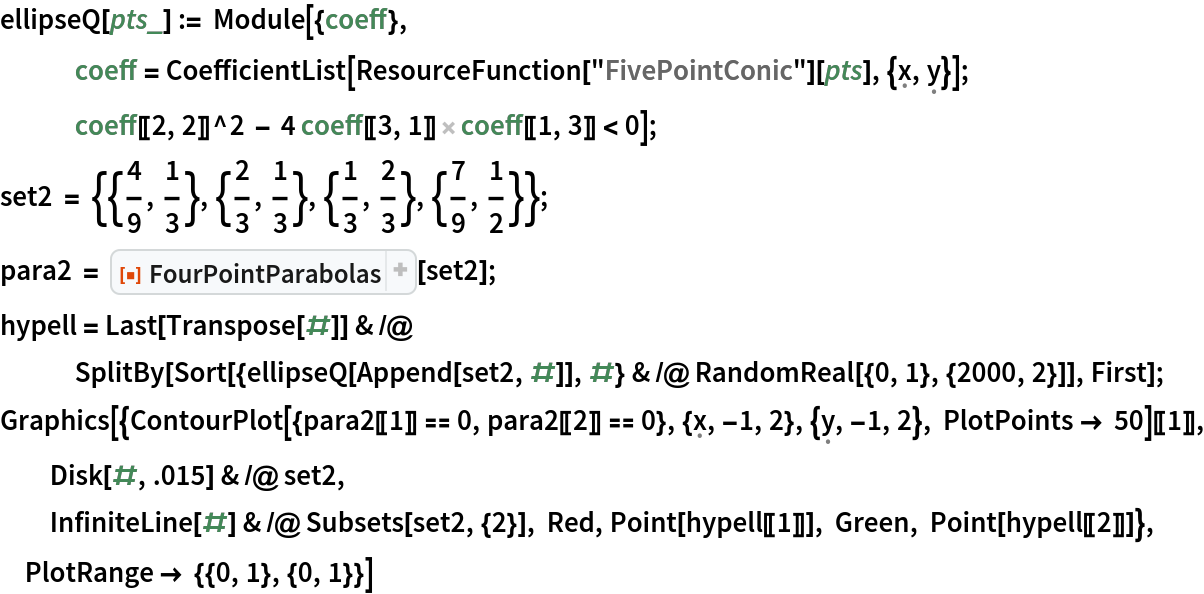Out=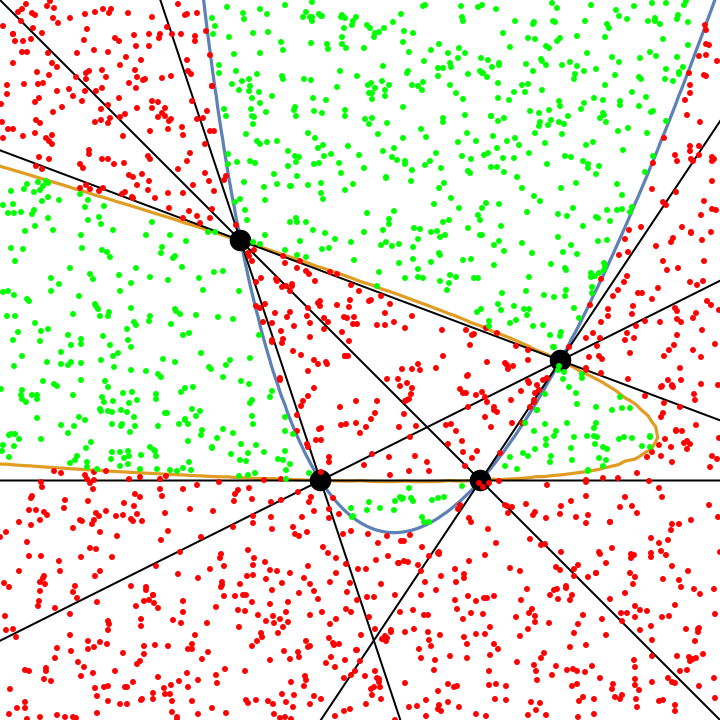Find the parabolas through a particular set of points on the regular 13-gon:

 In:=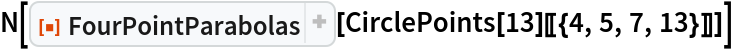Out=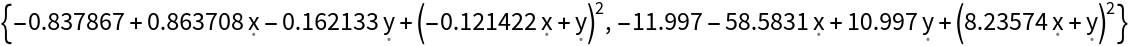Show the order four projective plane with parabolas:

 In:=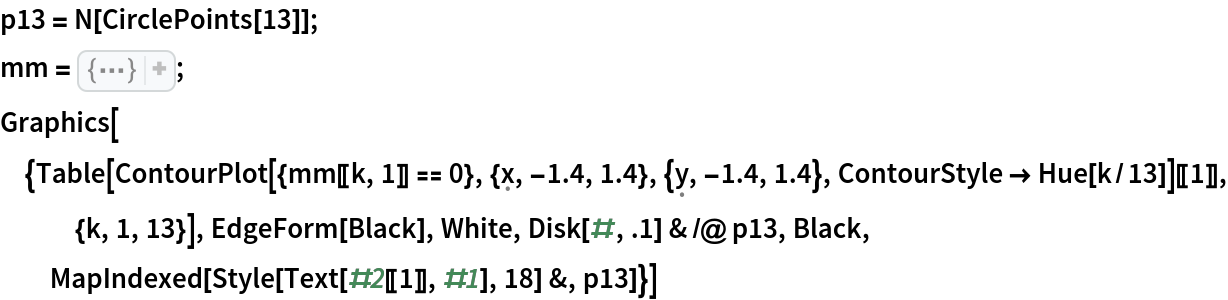Out=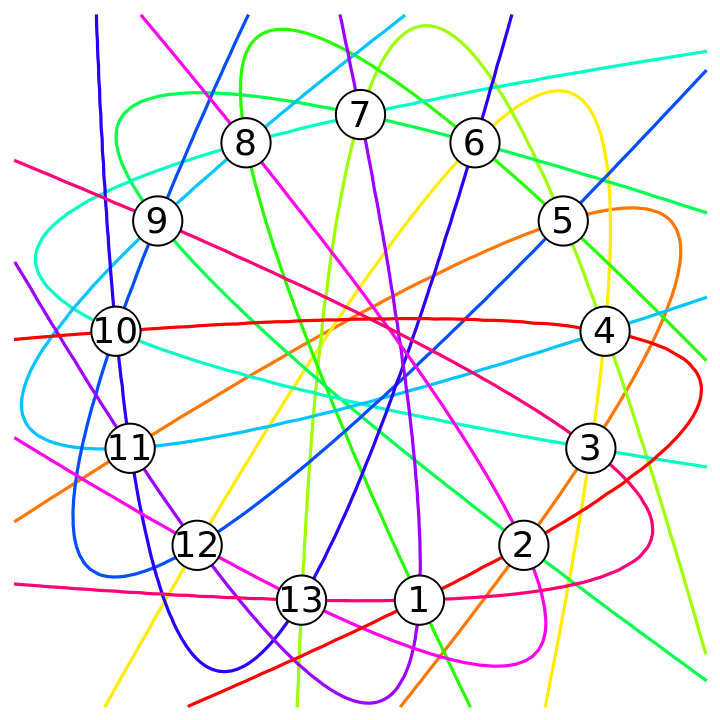Any pair of points defines exactly one parabola. Any pair of parabolas (and the unit circle) defines exactly one point.

## Version History

• 1.1.0 – 09 December 2022
• 1.0.0 – 02 December 2022